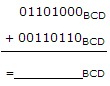# Digital Electronics - Digital Arithmetic Operations and Circuits

### Exercise :: Digital Arithmetic Operations and Circuits - General Questions

31.

A half-adder circuit would normally be used each time a carry input is required in an added circuit.

 A. True B. False

Answer: Option B

Explanation:

No answer description available for this question. Let us discuss.

32.

If B[7..0] = 10100101, what is the value of B[6..2]?

 A. 10100 B. 01001 C. 10010 D. 00101

Answer: Option B

Explanation:

No answer description available for this question. Let us discuss.

33.

How many inputs must a full-adder have?

 A. 4 B. 2 C. 5 D. 3

Answer: Option D

Explanation:

No answer description available for this question. Let us discuss.

34.A. 10011110 B. 01211110 C. 000100000100 D. 001000001000

Answer: Option C

Explanation:

No answer description available for this question. Let us discuss.

35.

Determine the two's-complement of each binary number.
00110        00011        11101

 A. 11001    11100    00010 B. 00111    00010    00010 C. 00110    00011    11101 D. 11010    11101    00011

Answer: Option D

Explanation:

No answer description available for this question. Let us discuss.

#### Current Affairs 2021

Interview Questions and Answers